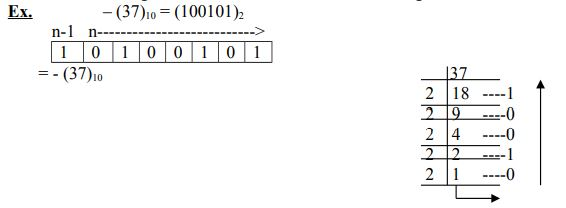### Ticker

6/recent/ticker-posts

# Number System Conversion in Computer

 S. No Name of Number system Digit Base/Radix Example 1 Binary 0,1 2 01110011 2 Decimal 0-9 10 89 3 Octal 0-7 8 176 4 Hexa-decimal 0-15, 0-F 16 7D

 Decimal Hexa-decimal Binary Octal 0 0 0000 0 1 1 0001 1 2 2 0010 2 3 3 0011 3 4 4 0100 4 5 5 0101 5 6 6 0110 6 7 7 0111 7 8 8 1000 9 9 1001 A 1010 B 1011 C 1100 D 1101 E 1110 F 1111

Sign bit: - “0 means +” and “1 means -". An integer can be positive or negative. We do not have any other symbol in the binary symbol. If sing bit is 0 then value will be positive or bit is 1 then value will be negative.

Signed magnitude scheme: -

Method: -

Step 1- Convert the decimal no. into its binary equivalent.

Step-2:- Represent the converted Binary no. in the no. of bits available for representation. If n bits are available for storing a decimal no. n-1 are used for the magnitude and 1 bits for sing bit.Convert the decimal no. into its binary equivalent.

### One’s compliments:-

One’s compliments is used to represent negative integer. Step Ist: convert the positive integer in the given no. of bits the sign bit will be 0 now. Step IInd: Flip all the bits that is convert “0s” into 1 and 1s into 0s.

ASCII: - The ASCII code used 7 bits to represent any character set. Earlier every manufacturer used a different code to represent a character resulting in choose and utter confusion. Finally the computer industry has accepted the ASCII code. The full form of ASCII is American Standard code for Information Interchange.

EBCDIC: - It is an 8 bit code. Large computer system uses this EBCDID code. The full form of EBCDIC is Extended Binary Coded Decimal Interchange Code.

Positive and negative integer: - the set of whole number is called set of integer. Thus the number having no fractional part and hence no decimal point are called integer. The positive integer are written without a sign before them where as negative integer having a (-) sing before them.

Floating point number: - In modern computer a real number is stored in he standard floating point form. A real number is set to be in standard floating point form if it is expressed as m*bn where b is the base of number system and n is the meatisaw which satisfy the relation. Ex. 0.111*2-13 are in standard floating point form.

ALSO SEARCH:

"number system conversion exercises with solutions"

"conversion of number system in computer"

"number system conversion"

"number system conversion calculator"

"what is the use of number system in computer"

"number system conversion in computer fundamentals"

"binary number system in computer"